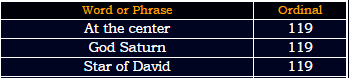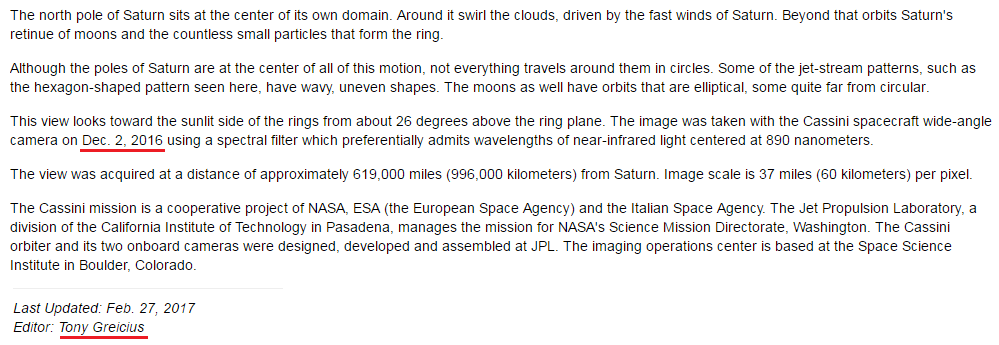CipherMar 2, 2017

2/27 Saturn Tribute from NASA, how unexpected

Link to the article - https://www.nasa.gov/image-feature/jpl/pia20520/at-the-center

This story was released on 27th of February (2/27) a number constantly showing up with Saturn coding and it's expected/appropriate to have a story about Saturn coming on this date"Saturn" = 45+1+20+21+18+14 = 119 (Francis Bacon)119 title on 2/27
119+191+911 = 1221
227+272+722 = 1221 (Saturn rules Capricorn from 12/21 to 1/19)Date numerology
2/27/2017 - 2+2+7 + 2+0+1+7 = 21 - Saturn = 1+1+2+3+9+5 = 21 (Reduced)
2/27/2017 - 2+27+ 2+0+1+7 = 39 - Saturn = 19+1+20+21+18+14 = 93 (Ordinal) also there's 39 books in the Old Testament which is clearly based around Saturn worship
2/27/2017 - 2+27+20+17 = 66 - editor of the story - Tony Greicius = 2+6+5+7 + 7+9+5+9+3+9+3+1 = 66 (Reduced)"Thirty three" = 2+8+9+9+2+7 + 2+8+9+5+5 = 66 (Reduced)

I feel like this Tony Greicius is in charge of coding Saturn for NASA, I see this name in almost every Saturn decode, Tony Greicius released a about the Moon of Saturn on 1/19 which is 39 days before 2/27

The "picture" was taken by "Cassini" = 3+1+10+10+9+5+9 = 47 (S Exception)
Matching with gematria of the title - "At the center" = 1+2 + 2+8+5 + 3+5+5+2+5+9 = 47 (Reduced)
"Cassini" = 24+26+8+8+18+13+18 = 115 (Reverse Ordinal)
"Saturn" = 90+1+100+200+80+40 = 511 (Jewish)
This "picture" was supposedly taken on "December Second" = 4+5+3+5+13+2+5+18 + 19+5+3+15+14+4 = 115 (Ordinal)

From the time "picture" was taken to 27th of February is 2 months and 26 days"Planet" = 60+20+1+40+5+100 = 226 (Jewish)
"Rings" = 80+9+40+7+90 = 226 (Jewish)
"Two hundred twenty six" = 2+5+6 + 8+3+5+4+9+5+4 + 2+5+5+5+2+7 + 1+9+6 = 93 (Reduced)
"Saturn" = 19+1+20+21+18+14 = 93 (Ordinal)

NASA truly couldn't be more predictable.....